## 长安福特 锐界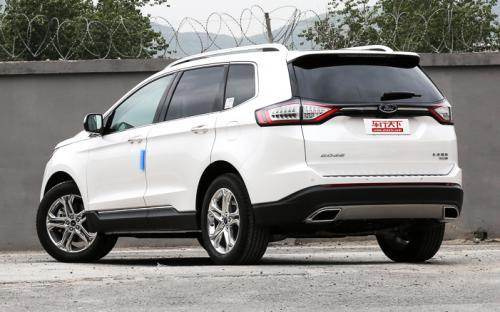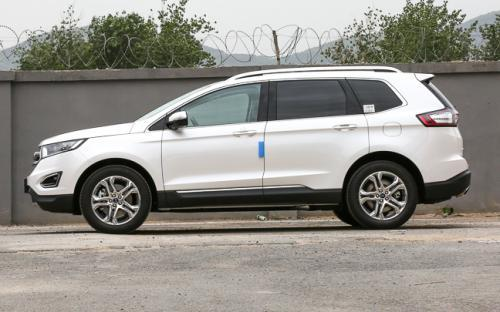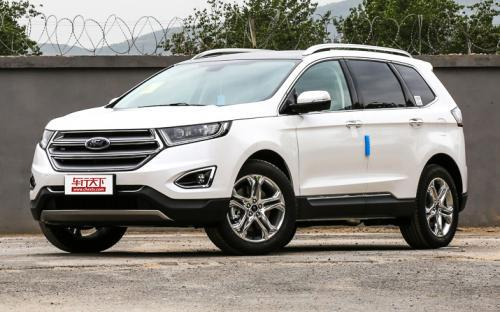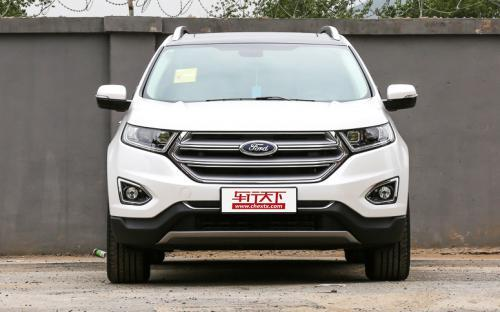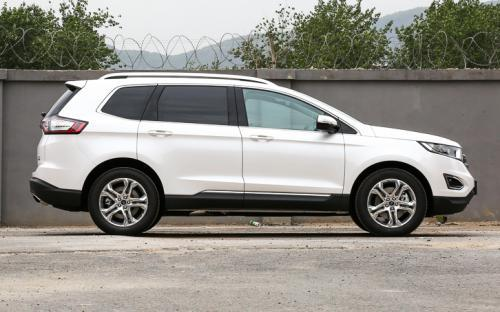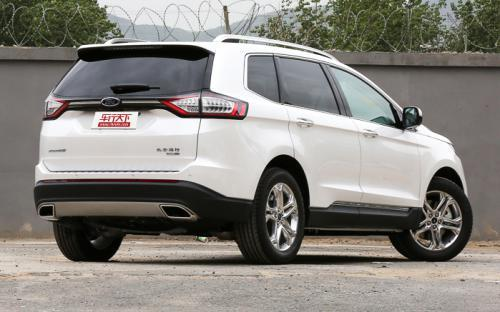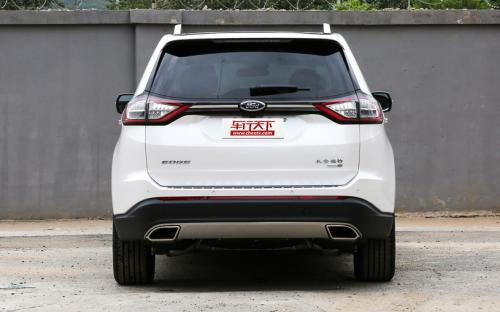7 种颜色可选2016款最低售价：24.98 万元起

4878(mm)1925(mm)1734(mm)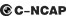##### 配置亮点：
• 胎压监测装置

• ISOFIX儿童座椅接口

• 车身稳定控制(ESC/ESP/DSC等)

• 电动天窗

• 定速巡航

• 后倒车雷达

• 真皮座椅

• GPS导航系统

• 氙气大灯

• 后视镜加热

2016款 EcoBoost 245 两驱精锐型 (138张)
• 2016款 EcoBoost 245 两驱精锐型 (138张)
• 2016款 EcoBoost 245 两驱豪锐型 (146张)
• 2016款 EcoBoost 245 四驱豪锐型 (160张)长安福特 锐界 在售车型

排量 车型 厂商指导价 本地最低报价 购车工具
2.0T EcoBoost 245 两驱精锐型 6挡手自一体 24.98万 24.98万

询底价+对比
EcoBoost 245 两驱铂锐型 6挡手自一体 27.98万 27.98万

询底价+对比
EcoBoost 245 两驱豪锐型 6挡手自一体 29.98万 询底价+对比
EcoBoost 245 四驱豪锐型 6挡手自一体 31.98万 询底价+对比
EcoBoost 245 四驱尊锐型 6挡手自一体 33.98万 33.98万

询底价+对比
2.7T EcoBoost 245 两驱精锐型 6挡手自一体 24.98万 24.98万

询底价+对比
EcoBoost 245 两驱铂锐型 6挡手自一体 27.98万 27.98万

询底价+对比
EcoBoost 245 两驱豪锐型 6挡手自一体 29.98万 询底价+对比
EcoBoost 245 四驱豪锐型 6挡手自一体 31.98万 询底价+对比
EcoBoost 245 四驱尊锐型 6挡手自一体 33.98万 33.98万

询底价+对比

长安福特 锐界 经销商

查看更多 >>
更多 >>

本地降价排名

### 长安福特 锐界 动力加速

锐界 0-100公里加速时间分布在 7.5-10秒 属于 运动级

动力级别 加速时间 车型
运动级(2款) 8.9s 2.0T GTDi 两驱精锐型
7.5s 2.7T GTDi 四驱运动型2.7T GTDi 四驱尊锐型
民用级(3款) 9.1s 2.0T GTDi 两驱铂锐型2.0T GTDi 两驱豪锐型
10.0s 2.0T GTDi 四驱豪锐型
9.5s 2.0T GTDi 四驱尊锐型

长安福特 锐界 视频

长安福特 锐界 新闻资讯

# 家用车选第四代胜达、锐界还是汉兰达？

试驾评测 超过11705次关注

由于“中国路况”以及家庭人口较多等方面的因素，SUV受到中国人民的追捧，我们选取北京现代第四代胜达、长安福特锐界、广汽丰田汉兰达,比较热门的三款SUV车型进行多...

# 第四代胜达凭实力说话的SUV

国产新车 超过14840次关注

在众多SUV中，春节出行到底选谁好呢？聚焦车市多种品牌多种车型，我们挑选了两款车型进行介绍，分别是北京现代第四代胜达与长安福特锐界。

# 第四代胜达对比锐界

试驾评测 超过9061次关注

SUV车型越来越被大众认可和追捧。北京现代第四代胜达和长安福特锐界作为今年的两款代表SUV车型，在这些方面的表现如何，又是哪一个更胜一筹呢？

# 长安福特新款锐界上市 售价24.98-33.98万

上市新车 超过9376次关注

5月23日，长安福特官方宣布2016款锐界正式上市，新车在外观方面没有发生改变，提供包含5座、7座版在内的5款2.0T车型，售价为24.98-33.98万元。

猜你喜欢

﻿
• 快速找车
• 选择品牌
• 选择品牌
• A  奥迪
• A  阿斯顿·马丁
• A  阿尔法·罗密欧
• B  宝沃
• B  布加迪
• B  巴博斯
• B  保时捷
• B  宾利
• B  奔驰
• B  宝马
• B  本田
• B  别克
• B  标致
• B  比亚迪
• B  宝骏
• B  北汽制造
• B  北汽新能源
• B  北汽幻速
• B  北汽威旺
• B  北京汽车
• B  奔腾
• B  北汽绅宝
• C  长安
• C  长安商用
• C  长城
• C  昌河
• D  大众
• D  道奇
• D  DS
• D  东南
• D  东风风神
• D  东风风行
• D  东风小康
• D  东风风度
• D  东风
• F  福特
• F  丰田
• F  菲亚特
• F  法拉利
• F  福田
• F  福迪
• F  福汽启腾
• G  观致
• G  广汽传祺
• G  广汽吉奥
• G  GMC
• H  红旗
• H  汉腾汽车
• H  哈弗
• H  哈飞
• H  海格
• H  海马
• H  华颂
• H  黄海
• H  华泰
• H  恒天
• J  吉利汽车
• J  捷豹
• J  Jeep
• J  江淮
• J  江铃
• J  金杯
• J  九龙
• J  金旅
• K  凯翼
• K  凯迪拉克
• K  克莱斯勒
• K  科尼塞克
• K  卡威
• K  开瑞
• L  路虎
• L  林肯
• L  劳斯莱斯
• L  兰博基尼
• L  雷克萨斯
• L  铃木
• L  雷诺
• L  理念
• L  力帆
• L  莲花汽车
• L  猎豹
• L  路特斯
• L  陆风
• M  马自达
• M  MG
• M  MINI
• M  玛莎拉蒂
• M  摩根
• M  迈凯轮
• N  纳智捷
• O  欧宝
• O  讴歌
• O  欧朗
• Q  奇瑞
• Q  起亚
• Q  启辰
• R  日产
• R  荣威
• R  瑞麒
• S  三菱
• S  斯威汽车
• S  萨博
• S  smart
• S  斯柯达
• S  斯巴鲁
• S  思铭
• S  双龙
• S  上汽大通
• S  双环
• T  特斯拉
• T  腾势
• W  沃尔沃
• W  五菱汽车
• W  五十铃
• W  威兹曼
• W  威麟
• X  现代
• X  雪佛兰
• X  雪铁龙
• X  西雅特
• Y  一汽
• Y  英菲尼迪
• Y  英致
• Y  依维柯
• Y  野马汽车
• Y  永源
• Z  众泰
• Z  中华
• Z  中兴
• Z  知豆
• 选择车系
• 选择车系
• 车型对比
• 选择品牌
• 选择品牌
• A  奥迪
• A  阿斯顿·马丁
• A  阿尔法·罗密欧
• B  宝沃
• B  布加迪
• B  巴博斯
• B  保时捷
• B  宾利
• B  奔驰
• B  宝马
• B  本田
• B  别克
• B  标致
• B  比亚迪
• B  宝骏
• B  北汽制造
• B  北汽新能源
• B  北汽幻速
• B  北汽威旺
• B  北京汽车
• B  奔腾
• B  北汽绅宝
• C  长安
• C  长安商用
• C  长城
• C  昌河
• D  大众
• D  道奇
• D  DS
• D  东南
• D  东风风神
• D  东风风行
• D  东风小康
• D  东风风度
• D  东风
• F  福特
• F  丰田
• F  菲亚特
• F  法拉利
• F  福田
• F  福迪
• F  福汽启腾
• G  观致
• G  广汽传祺
• G  广汽吉奥
• G  GMC
• H  红旗
• H  汉腾汽车
• H  哈弗
• H  哈飞
• H  海格
• H  海马
• H  华颂
• H  黄海
• H  华泰
• H  恒天
• J  吉利汽车
• J  捷豹
• J  Jeep
• J  江淮
• J  江铃
• J  金杯
• J  九龙
• J  金旅
• K  凯翼
• K  凯迪拉克
• K  克莱斯勒
• K  科尼塞克
• K  卡威
• K  开瑞
• L  路虎
• L  林肯
• L  劳斯莱斯
• L  兰博基尼
• L  雷克萨斯
• L  铃木
• L  雷诺
• L  理念
• L  力帆
• L  莲花汽车
• L  猎豹
• L  路特斯
• L  陆风
• M  马自达
• M  MG
• M  MINI
• M  玛莎拉蒂
• M  摩根
• M  迈凯轮
• N  纳智捷
• O  欧宝
• O  讴歌
• O  欧朗
• Q  奇瑞
• Q  起亚
• Q  启辰
• R  日产
• R  荣威
• R  瑞麒
• S  三菱
• S  斯威汽车
• S  萨博
• S  smart
• S  斯柯达
• S  斯巴鲁
• S  思铭
• S  双龙
• S  上汽大通
• S  双环
• T  特斯拉
• T  腾势
• W  沃尔沃
• W  五菱汽车
• W  五十铃
• W  威兹曼
• W  威麟
• X  现代
• X  雪佛兰
• X  雪铁龙
• X  西雅特
• Y  一汽
• Y  英菲尼迪
• Y  英致
• Y  依维柯
• Y  野马汽车
• Y  永源
• Z  众泰
• Z  中华
• Z  中兴
• Z  知豆
• 选择车系
• 选择车系
• 选择车型
• 选择车型
• 意见反馈